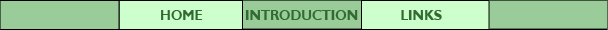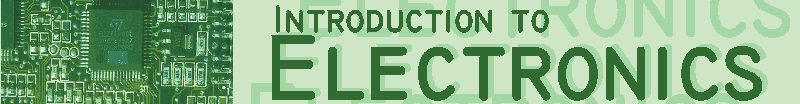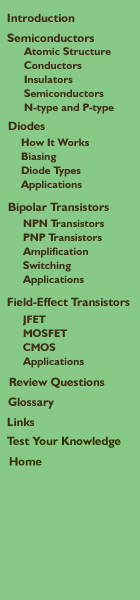# Review Questions

1. Using the Bohr model of atomic structure, explain the differences between conductors and insulators.

2. Why are silicon and germanium referred to as "semiconductors"?

3. What is the difference between N-type and P-type semiconductor?

4. The symbol below represents the device called a ____________.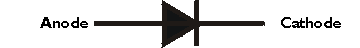5. List three types of diodes and explain what they are used for.

6. The bipolar transistor is a three-layer semiconductor device. Label the three parts on the diagram below.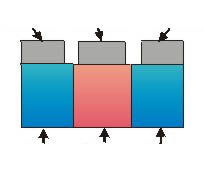7. The three layers of the bipolar transistor can be arranged in two ways.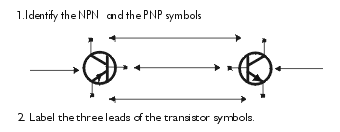8. Transistors have two main applications. Name them.

9. Complete the following statement:
In a transistor circuit, the current through the _____________________ is
many times greater than the __________________ current.

10. Explain how the operation of Field Effect Transistors differs from that of Bipolar Junction Transistors.

11. A ) What does the acronym MOSFET stand for?

B) What material is used to insulate the gate terminal from the conductive channel in a MOSFET?

C) Draw a diagram of a MOSFET indicating the direction of electron flow when the device is conducting. Label all the terminals.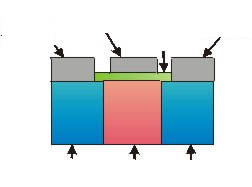12. Why is CMOS technology used in microprocessors?

13. Before the invention of the transistor, vacuum tubes and relays performed a similar function; what advantages do transistors have over earlier technologies?

14. Write the name of each device shown in the space provided.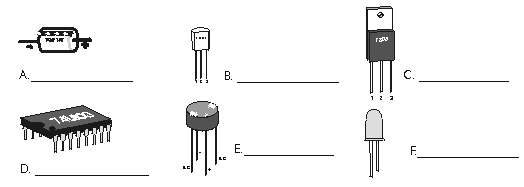#### Multiple Choice

1. No current flows in a transistor when it is:
A) Cut-off
B) Saturated
C) Amplifying
D) Forward-biased

2. The transistor amplifies if it is operating in which of the following bias regions:
A) Cut-off
B) Saturation
C) Linear
D) Reverse-biased

3. A transistor, when used in digital applications, acts like a(n):
A) amplifier
B) oscillator
C) variable resistor
D) switch